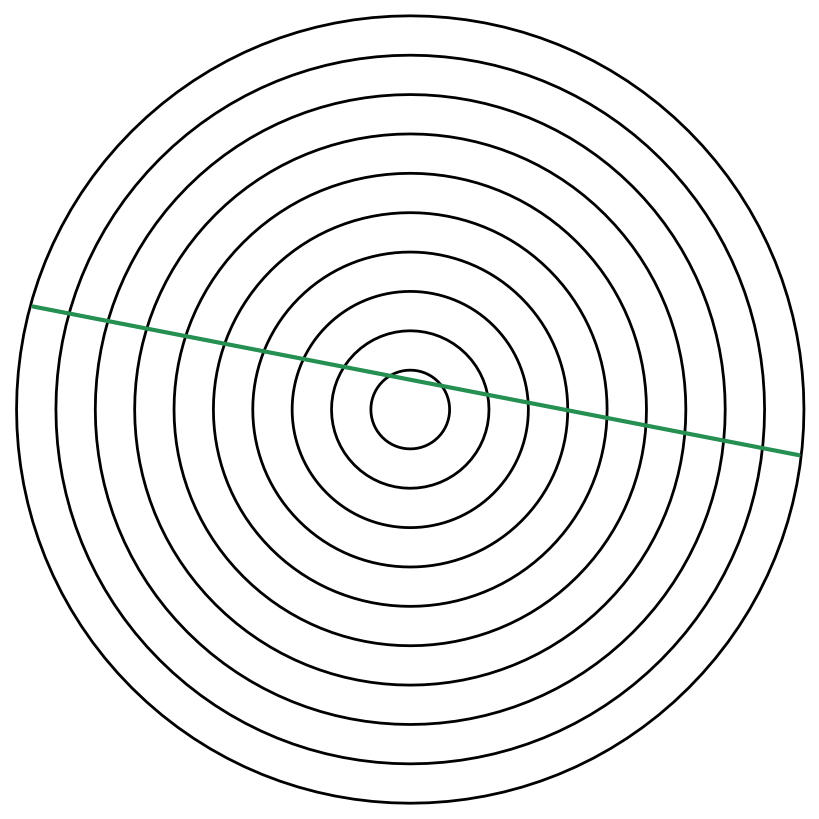# Concentric Circles Equally Split This Chord

Geometry Level 5Let $[A]$ denote the area of circle $A$. Suppose that $10$ concentric circles $O_1,O_2,\ldots,O_{10}$ satisfy $[O_i] > [O_{i+1}]$ for all $i=1\to 9$. Also, a chord drawn in circle $O_1$ has the property that circles $O_2\to O_{10}$ cut it into $19$ equal sections. The chord has length $2014$, and $[O_1]+[O_2]+[O_3]+\cdots +[O_{10}]<20140000$

What is the largest possible integer value of the radius of $O_{10}$?

You are permitted to use a scientific calculator.

×